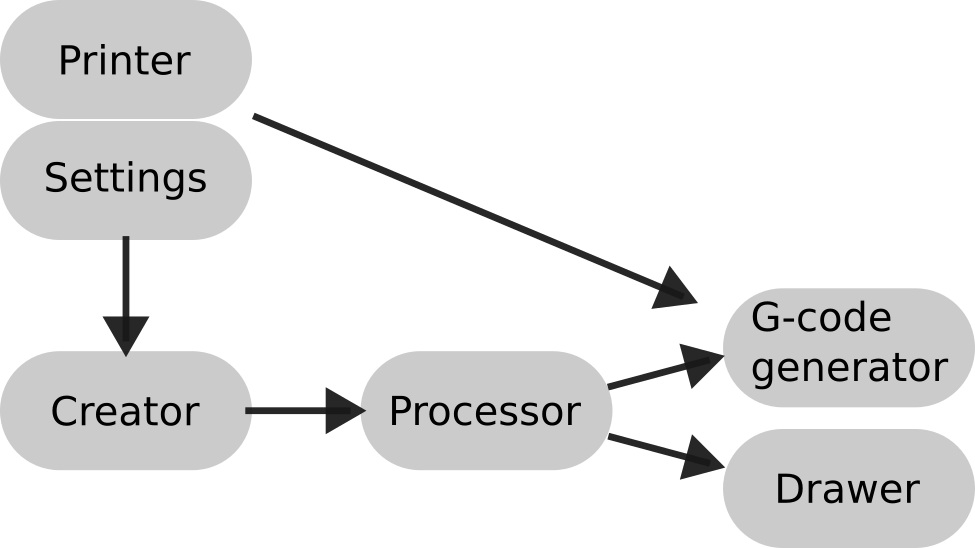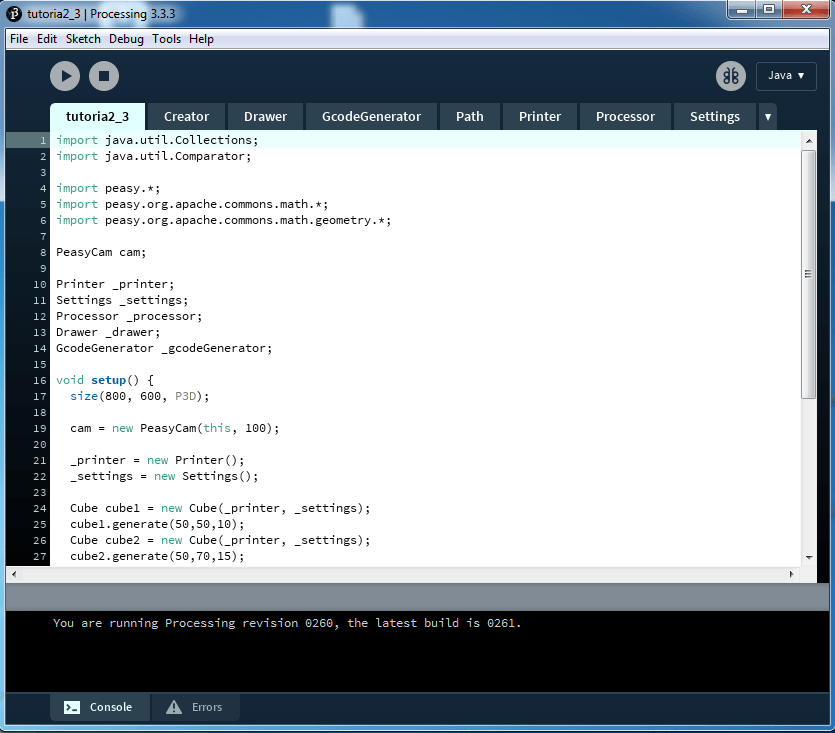# Learn to code: Make G-code with Processing (Part 2)

In the previous tutorial we have seen how to simply generate G-code with Processing. We will now continue on this track, developing a more robust framework for our experiments using Object Oriented Programming (OOP). Let’s see how we can do that.

## Program structure

First of all we need to think of a structure for our program. We need something that is simple and yet robust and flexible enough, so that we can reuse it in the future. This is how we will do it, implementing the following classes.We will create two classes that will host the settings of our program, one related to the printer (for example the maximum printing volume) and one for the printing settings (path with, layer height, speed etc.).

There will then be a creator class. It will create the paths that will be used to generate the G-code. What we generate will depend on what we have in mind, what objects we want to create. This is the part of the code that we will rewrite in different projects, while the rest, if we do things correctly, will remain the same.

The creator class will then go into a processor class that will prepare the paths previously generated for the G-code generation and for the visualization.

The last two classes are, in fact, a G-code generator that will produce our file for printing and a drawer for visualization which we will link in a second moment to a GUI.

Let’s start writing the code.

## The code

First, the `Printer` class:

```class Printer {
// Default setting for ZMorph 2SX
float width_table = 235; //mm
float length_table = 250; //mm
float height_printer = 165; //mm

float x_center_table = width_table / 2.0f;
float y_center_table = length_table / 2.0f;
}```

Then, the `Settings `class:

```class Settings {
float path_width = 0.4; //mm
float layer_height = 0.2; //mm
float filament_diameter = 1.75; //mm

float default_speed = 1500; //mm/minute
float travel_speed = 3000; //mm/minute

int start_fan_at_layer = 3;

float extrusion_multiplier = 3;

float retraction_amount = 4.5; //mm
float retraction_speed = 5000; //mm/minute

float getExtrudedPathSection(){
return path_width * layer_height; //mm^2
}

float getFilamentSection(){
return PI * sq(filament_diameter/2.0f); //mm^2
}
}```

Now let’s write a `Path `class which will represent the extrusion movements of our printer. The `getCenter()` method will be used by the `Processor `class.

```class Path {
ArrayList<PVector> vertices;

Path() {
vertices = new ArrayList<PVector>();
}

}

PVector getCenter() {

float mean_X = 0, mean_Y = 0, mean_Z = 0;

for (PVector p : vertices) {
mean_X += p.x;
mean_Y += p.y;
mean_Z += p.z;
}

mean_X = mean_X / vertices.size();
mean_Y = mean_Y / vertices.size();
mean_Z = mean_Z / vertices.size();

PVector center = new PVector(mean_X, mean_Y, mean_Z);

return center;
}
}```

For the `Creator` we will use this approach: first a generic class;

```class Creator {
ArrayList<Path> paths = new ArrayList<Path>();

Printer printer;
Settings settings;

Creator(Printer t_printer, Settings t_settings) {
printer = t_printer;
settings = t_settings;
}
}```

Now, by inheritance, we can create all the children that we want, as far as they fill the `paths `ArrayList. This is where you can get creative and write all sorts of algorithms for generating the shapes that you have in mind. Now, just as an example, let’s write a class that will generate the paths for our usual cube:

```class Cube extends Creator {

Cube(Printer t_printer, Settings t_settings) {
super(t_printer, t_settings);
}

void generate(float c_x, float c_y, float length_side_cube) {

paths = new ArrayList<Path>();

float tot_layers = length_side_cube / settings.layer_height;
float angle_increment = TWO_PI / 4.0f;
float z = 0;

for (int layer = 0; layer<tot_layers; layer++) {

z += settings.layer_height;

for (float angle = 0; angle<=TWO_PI; angle+=angle_increment) {

float x = c_x + cos(angle) * length_side_cube;
float y = c_y + sin(angle) * length_side_cube;

PVector next_point = new PVector(x, y, z);

}
}
}
}```

The constructor of the `Cube `is the same as for its parent. We then have a `generate()` function that accepts as parameters the position of the cube on the table and its size.

This structure allows us to create multiple objects (`Creator `classes). However, in order to generate the G-code, we need to put all the paths of the objects together and give them some order. This is what the `Processor `class does. Let’s look at it step by step:

```class Processor {

ArrayList<Creator> objects = new ArrayList<Creator>();
ArrayList<Path> paths;

return this;
}
}```

As we can see, the `Processor `hosts an ArrayList of `Creators `called `objects`. We can add a new object through  the method `addObject()`. There is then a field called `paths`: this is what will be read by the class for G-code generation and by the one for visualization. What we want to do now is fill this ArrayList. We will put all the objects’path together and we will then sort them from bottom to top (following the extrusion order). We will do all of this in one method called `sortPaths()`:

```void sortPaths() {
paths = new ArrayList<Path>();

//Put all the outlines of the objects in one ArrayList

for (Creator obj : objects) {
for (Path out : obj.paths) {
}
}

//Sort them from bottom to top layer

Collections.sort(paths, new Comparator<Path>() {
public int compare(Path o1, Path o2) {
return Float.compare(o1.getCenter().z, o2.getCenter().z);
}
}
);
}```

Regarding the second part of the method, we could have written a custom sorting algorithm, however since Processing is based on Java, we can take advantage of the built in sort algorithms of the language, you just need add `import java.util.Collections;` and `import java.util.Comparator;` to your program.

Now we have what we need to generate our G-code. Let’s then write the `GcodeGenerator `class. The constructors takes as parameters the `Printer `class, the `Settings `class and the `Processor `class. it uses the paths from the `Processor `to generate the paths for the printer. This is done inside the `generate()` method. All the other methods are based on what we have seen in the previous tutorials and you should not have problems to understand them. Note that we have changed the `endPrint()` method so that now it is related to the `Printer` object parameters.

```class GcodeGenerator {

ArrayList<String> gcode;
Printer printer;
Settings settings;
Processor processor;

float E = 0; // Left extruder

GcodeGenerator(Printer t_printer, Settings t_settings, Processor t_processor) {
printer = t_printer;
settings = t_settings;
processor = t_processor;
}

GcodeGenerator generate() {
gcode = new ArrayList<String>();

float extrusion_multiplier = 1;

startPrint();

for (Path path : processor.paths) {

moveTo(path.vertices.get(0));

if (getLayerNumber(path.vertices.get(0)) < settings.start_fan_at_layer) {
setSpeed(settings.default_speed/2);
} else if (getLayerNumber(path.vertices.get(0)) == settings.start_fan_at_layer) {
setSpeed(settings.default_speed);
enableFan();
} else {
setSpeed(settings.default_speed);
}

extrusion_multiplier = getLayerNumber(path.vertices.get(0)) == 1 ? settings.extrusion_multiplier : 1;

for (int i=0; i<path.vertices.size()-1; i++) {
PVector p1 = path.vertices.get(i);
PVector p2 = path.vertices.get(i+1);
extrudeTo(p1, p2, extrusion_multiplier);
}
}

endPrint();

return this;
}

int getLayerNumber(PVector p) {
return (int)(p.z/settings.layer_height);
}

void write(String command) {
}

void moveTo(PVector p) {
retract();
write("G1 " + "X" + p.x + " Y" + p.y + " Z" + p.z + " F" + settings.travel_speed);
recover();
}

float extrude(PVector p1, PVector p2) {
float points_distance = dist(p1.x, p1.y, p2.x, p2.y);
float volume_extruded_path = settings.getExtrudedPathSection() * points_distance;
float length_extruded_path = volume_extruded_path / settings.getFilamentSection();
return length_extruded_path;
}

void extrudeTo(PVector p1, PVector p2, float extrusion_multiplier) {
E+=(extrude(p1, p2) * extrusion_multiplier);
write("G1 " + "X" + p2.x + " Y" + p2.y + " Z" + p2.z + " E" + E);
}

void extrudeTo(PVector p1, PVector p2, float extrusion_multiplier, float f) {
E+=(extrude(p1, p2) * extrusion_multiplier);
write("G1 " + "X" + p2.x + " Y" + p2.y + " Z" + p2.z + " E" + E + " F" + f);
}

void retract() {
E-=settings.retraction_amount;
write("G1" + " E" + E + " F" + settings.retraction_speed);
}

void recover() {
E+=settings.retraction_amount;
write("G1" + " E" + E + " F" + settings.retraction_speed);
}

void setSpeed(float speed) {
write("G1 F" + speed);
}

void enableFan() {
write("M 106");
}

void disableFan() {
write("M 107");
}

void startPrint() {
write("G91"); //Relative mode
write("G1 Z1"); //Up one millimeter
write("G28 X0 Y0"); //Home X and Y axes
write("G90"); //Absolute mode
write("G1 X" + printer.x_center_table + " Y" + printer.y_center_table + " F8000"); //Go to the center
write("G28 Z0"); //Home Z axis
write("G1 Z0"); //Go to height 0
write("T0"); //Select extruder 1
write("G92 E0"); //Reset extruder position to 0
}

void endPrint() {
PVector last_position =           processor.paths.get(processor.paths.size()-1).vertices.get(processor.paths.get(processor.paths.size()-1).vertices.size()-1);

retract(); //Retract filament to avoid filament drop on last layer

//Facilitate object removal
float end_Z;
if (printer.height_printer - last_position.z > 10) {
end_Z = last_position.z + 10;
} else {
end_Z = last_position.z + (printer.height_printer - last_position.z);
}
moveTo(new PVector(printer.x_center_table, printer.length_table - 10, end_Z));

recover(); //Restore filament position
write("M 107"); //Turn fans off
}

void export() {
//Create a unique name for the exported file
String name_save = "gcode_"+day()+""+hour()+""+minute()+"_"+second()+".g";
//Convert from ArrayList to array (required by saveString function)
String[] arr_gcode = gcode.toArray(new String[gcode.size()]);
// Export GCODE
saveStrings(name_save, arr_gcode);
}
}

```

Last, let’s visualize what we have generated. We will also show the printing chamber, so that we can have an idea of where our objects are and how big they are. This will become important when we will add a GUI in the following tutorial.

```class Drawer {
Processor processor;
Printer printer;

Drawer(Processor t_processor, Printer t_printer) {
processor = t_processor;
printer = t_printer;
}

void display() {

showPrinterChamber();

for (Path path : processor.paths) {
for (int i=0; i< path.vertices.size()-1; i++) {
PVector p1 = path.vertices.get(i);
PVector p2 = path.vertices.get(i + 1);
line(p1.x, p1.y, p1.z, p2.x, p2.y, p2.z);
}
}
}

void display(color c) {
stroke(c);
display();
}

void showPrinterChamber() {
pushMatrix();
translate(printer.x_center_table, printer.y_center_table, 0);
fill(200);
stroke(0);
rectMode(CENTER);
rect(0, 0, printer.width_table, printer.length_table);
rectMode(CORNER);
translate(0, 0, printer.height_printer/2);
noFill();
box(printer.width_table, printer.length_table, printer.height_printer);
popMatrix();
}
}```

Great, now we have all the classes that we need. I suggest you to put each one of them inside a different tab of Processing, in order to keep the program tidy.Now, in the main tab, we can test what we have so far written. For 3D navigation we will use the Processing library PeasyCam. You can download it via Sketch/Import Library…/Add Library…. Run the sketch and you will see the two cubes on the screen and, if you open the sketch folder, the G-code file for printing.

```import java.util.Collections;
import java.util.Comparator;

import peasy.*;
import peasy.org.apache.commons.math.*;
import peasy.org.apache.commons.math.geometry.*;

PeasyCam cam;

Printer _printer;
Settings _settings;
Processor _processor;
Drawer _drawer;
GcodeGenerator _gcodeGenerator;

void setup() {
size(800, 600, P3D);

cam = new PeasyCam(this, 100);

_printer = new Printer();
_settings = new Settings();

Cube cube1 = new Cube(_printer, _settings);
cube1.generate(50,50,5);
Cube cube2 = new Cube(_printer, _settings);
cube2.generate(55,70,15);

_processor = new Processor();
_processor.sortPaths();

_drawer = new Drawer(_processor, _printer);

_gcodeGenerator = new GcodeGenerator(_printer, _settings, _processor);
_gcodeGenerator.generate().export();
}

void draw() {
background(255);

_drawer.display();
}```

Good, from now on we can start to get serious.

In the next tutorial we will write a program for vase generation with a GUI for interactively modify our creations. See you next time!